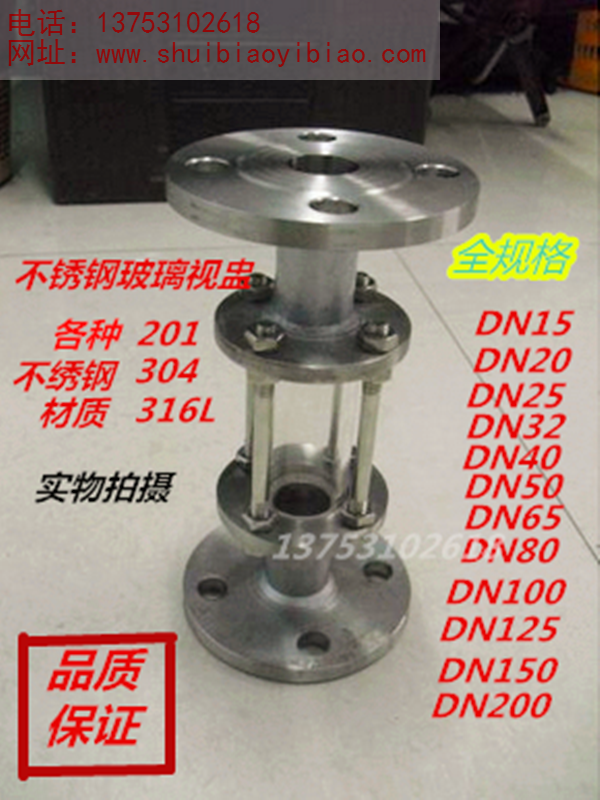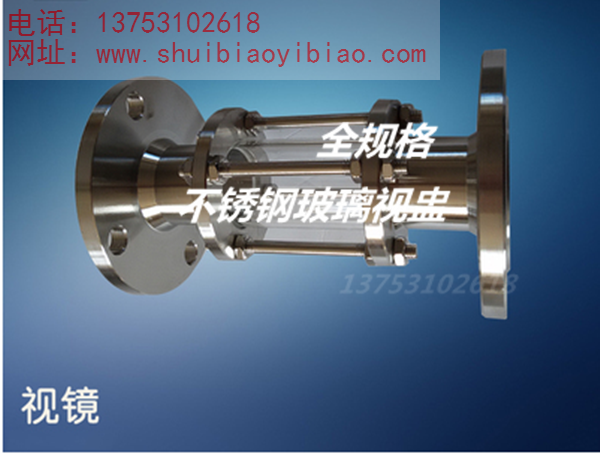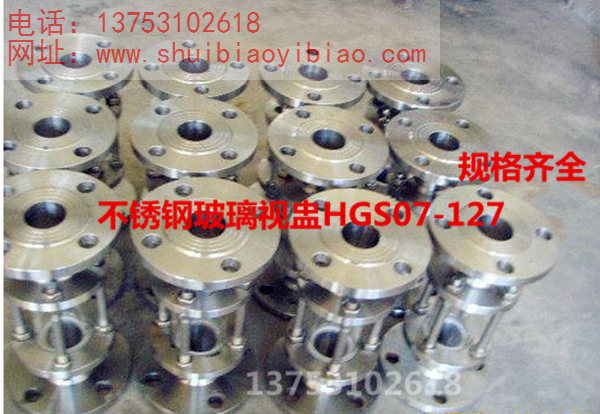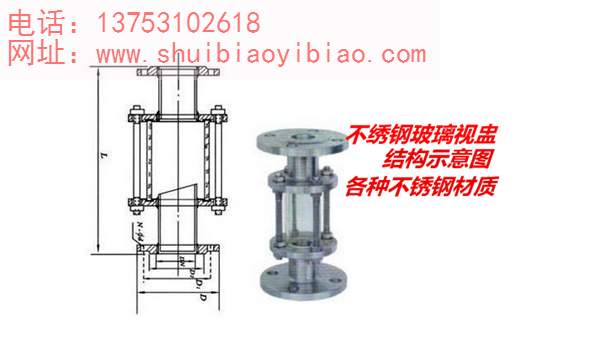### 产品中心

316L不锈钢法兰玻璃视盅 316L不锈钢法兰直通视盅DN15-DN200DN6=1/8=1分  DN8=1/4=2分  DN10=3/8=3分  DN15=1/2=4分N20=3/4=6分  DN25=1寸 DN32=1.2寸=1-1/4  DN40=1.5寸=1-1/2

DN50=2寸  DN65=2.5寸=2-1/2  DN80=3寸 DN100=4寸

⑴ 采用J44W-220R DN15高压截止阀形式，锥面密封，聚四氟乙烯填料密封。

⑵ 阀盖与阀体有螺栓连接，拆装方便⑷ 整体式阀体，双阀杆结构，外型布局合理。

⑶ 阀瓣与阀杆为整体结构，阀体内腔介质流道通畅，防止假液位。

⑷ 整体式阀体，双阀杆结构，外型布局合理。

⑸ 阀体可通保温蒸汽，作为介质保温，防止液体结晶。

⑹ 阀体与塔类容器焊接，提高阀体温度，二次防止液体结晶。

https://item.taobao.com/item.htm?spm=a1z10.1-c.w4004-13361780108.6.h8uZFy&id=534194346804